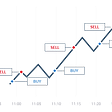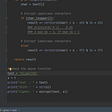# A Python tutorial to understand the relationship between Humidity and Temperature to using Machine Learning

Machine Learning has many applications in solving real world problems. Are these days feeling too warm for you? Is there a relationship between Humidity and Temperature?

To start this exercise, we will need a few tools. You must install Python programming language and set your environment variables correctly in order to proceed with the next steps. Then you must install Jupyter Notebook and download this dataset which consists of weather data.

https://www.kaggle.com/budincsevity/szegedweather?select=weatherHistory.csv

Now let’s proceed with the following steps before cleaning our data.

If not used before, it is essential to install scipy, pandas, sklearn, matplotlib and seaborn using pip. These are useful libraries to be used.

Import pandas library as below and load the csv file from the storage.

We need to ensure that the data we are using is free from missing values and outliers that will make us calculate messy test results.

If we use the head() function, we can see the data below.

Sometimes there maybe instances where there are empty value cells such as null or NaN. These must be removed as below but first we need to check if there are any null values using the isnull() function combined with any().

We can clearly see that only Precip Type has null values. If we use

df[‘Precip Type’].value_counts()

we get only Rain, Snow and of course null. Because rain is more than snow, we can replace the null values with rain as below but later in this tutorial, you will see that this particular column will not be very useful.

Once we have successfully removed the null values, we can remove the outliers if there are any.

Outliers are very large or very small values that appear infrequently and outside a boxplot range. These out of range values may be errors and we must remove them so that we can get more accurate predictions and have less variations among the available features.

To get a basic idea of the outliers, we use the boxplot() function.

As seen above, they are not very clear but we can visualize individual boxplots. Below is an example for one boxplot (Pressure column).

As seen above, there is an outlier where 0 is a very unnatural value (we’re all gonna die!!!)

So it is understandable that these can be calculation errors and therefore we must remove them as the following using a row by deletion.

Now we will get a boxplot as seen above. There are still outliers but we only delete the ones that are significant and not continuous as seen above. Further we repeat the same process for the Humidity and Windspeed columns.

Now we have a ‘cleaner’ dataset. Let’s move onto the standardization.

Here we look at plotting histograms and QQ plots in order to understand the distribution of our data. Then we apply transformation codes to change the distribution of the data if it is right skewed or left skewed.

As seen above, there are 2 histograms that are significantly skewed after using hist(). They are Humidity which is left skewed and Wind Speed which is right skewed. We need to transform these two features. We need to import scipy and matplotlib libraries for this.

The Wind Speed feature is right skewed. For this we use a logarithmic transformation by using the log transformation.

The Humidity feature is left skewed. For this we use a logarithmic transformation by using the exp transformation.

We import numpy and FunctionTransformer. It is best to drop the Loud Cover column since it only consists of 0s and this will create an error in the next step if not removed in this step.

With a new variable named data, we replace all the 0s with NaN using numpy. Then we will drop the NaN values with dropna() function. If we don’t remove Loud Cover, we will end up with an empty table since all rows will be deleted.

By creating two transformations, log transformation for right skew and exp transformation for left skew, we plus in the relevant columns to be transformed and assign it back to the data dataframe. If you run hist() again, you will see a result as below.

We can see a small change in the shape because of the standardization.

This dataset has one variable that can be used to demonstrate feature coding in order to give a numerical value to a discrete feature.

As clearly seen above, we can assign a dummy values for Precip Type and if it is rain, we get a 1–0 combination while it would be vice versa for snow.

Scaling is also known as z score normalisation. We are normalising the values to the standard deviation so that it will provide more accurate results in the later steps.

We import StandardScaler to perform scaling. We will only select columns that have continuous data. Once we fit the new data into the scaler model and convert it to a dataframe, we end up with values as below.

We don not need string data values and now we are only left with a dataframe of floating point values and all of the values are normalised, adjusted to their mean values and standard deviation. This will be use for Principal Component Analysis to follow.

Correlations are how well variables affect the behaviour of other variables. This leads us to the case study question, does humidity have a relationship with temperature. Let’s solve this question once and for all!

We need to import the seaborn library. We copy the scaled dataframe and we apply the above functions to create the correlation matrix table.

This colour coded heatmap you see above indicates the correlations of each variables. Theoretically speaking, you need values that are close to +1 or -1 in order to consider it as a strong correlation. We can also see values that are higher than -0.6 so we may consider it, even though the range -0.7 to -1 comes under the strong positively correlated range. Humidity shows a -0.6 plus correlation with temperature and apparent temperature, so we conclude that there is a relationship between the variables.

Furthermore, Temperature and Apparent Temperature have a +0.99 correlation which is very strong. This means that both variables should not be paired into the machine learning model but Apparent Temperature is what we want to predict so that will be our target variable. All other variables can be considered as significantly independent.

Now we do the Principal Component Analysis since we have some idea about the variables that are dependent and independent.

We import PCA and we list the features to be reduced in PCA and we exclude Apparent temperature because it is the target column.

As seen above, we get 6 columns and further by using the explained ratio variance, we get an array and the total is approximately 99, which means that we have extracted the significant features that have the most variance to the machine learning model.

We need to add back the Apparent Temperature column so we concatenate the dataframe components and get a result as above. We have almost reached the last step which is building the machine learning model. Again, we use the dropna() function to remove NaN values (otherwise the model won’t work).

Regression Model is a supervised Machine learning technique because we are comparing the inputs and predicted inputs that are generated using regression functions (line of best fit).

We import the relevant libraries from sklearn and we use the pop() function to include only Apparent Temperature values for Y and all the other columns except humidity to X.

We then assign variables to train and test each for X and Y. We use a 80 to 20 ratio to split the training and test data. To have an idea of what kind of data will be going as the input, we use head() for the train_X variable.

Once we use the LinearRegression() function and fit the training data, we make a prediction variable by assigning the test_X data and get the accuracy of our model as compared to the test_Y data.

In the end, we get a mean square error of 0.01 and a score of 99% which is very good.

Using Python and Jupyter Notebook, assisted by many useful libraries, we were able to find that Humidity and Temperature have a relationship and develop a machine learning model to predict Apparent Temperature.

The Python code that was used to do all the steps is given below.

--

--

--

Data Science enthusiast, journalist and public speaker. IT Undergraduate at University of Moratuwa

Love podcasts or audiobooks? Learn on the go with our new app.

## Ghosts From the Past, Part II## Let’s dance together! (Cool Dance Video Compilation)## Smart City Illustrations## How to prepare yourself for disappointment — Five Best Ideas of the Day: November 4, 2020## How to confuse future archeologists## Amusing Dalmations (Funny Dog Video Compilation)## Akhila Seneviratne

Data Science enthusiast, journalist and public speaker. IT Undergraduate at University of Moratuwa

## Imagination based AIs — Part -2## How to Backtest and Assess The Performance of Your Trading Strategy## Python Scripts for Traditional Cryptography